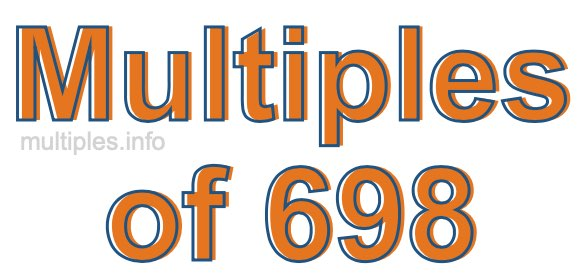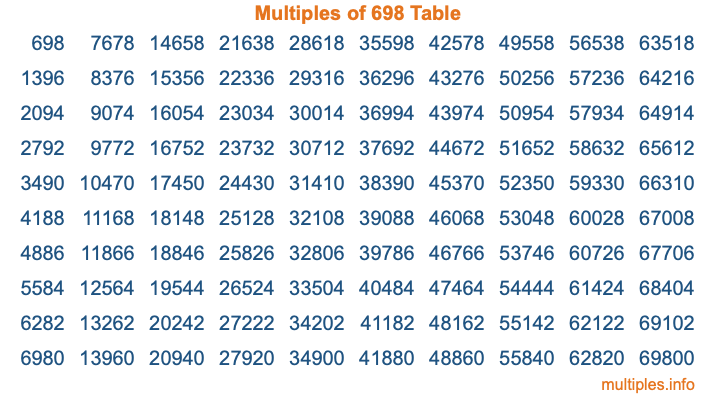Multiples of 698Welcome to the Multiples of 698 page. Here we will first teach you everything you will ever need to know about the multiples of 698, and then give you a study guide summary of everything we taught you to make sure you remember it all. Use this page to look up facts and learn information about the multiples of 698. This page will make you a multiples of six hundred ninety-eight expert!

Definition of Multiples of 698
Multiples of 698 are all the numbers that when divided by 698 equal an integer. Each of the multiples of 698 are called a multiple. A multiple of 698 is created by multiplying 698 by an integer.

Therefore, to create a list of multiples of 698, you start with 1 multiplied by 698, then 2 multiplied by 698, then 3 multiplied by 698, and so on for as long as you want. Thus, the list of the first five multiples of 698 is 698, 1396, 2094, 2792, and 3490. To see a larger list of multiples of 698, see the printable image of Multiples of 698 further down on this page. We also have a category where you can choose any nth multiple of 698.

Multiples of 698 Checker
The Multiples of 698 Checker below checks to see if any number of your choice is a multiple of 698. In other words, it checks to see if there is any number (integer) that when multiplied by 698 will equal your number. To do that, we divide your number by 698. If the the quotient is an integer, then your number is a multiple of 698.

Is  a multiple of 698?

Least Common Multiple of 698 and ...
A Least Common Multiple (LCM) is the lowest multiple that two or more numbers have in common. This is also called the smallest common multiple or lowest common multiple and is useful to know when you are adding our subtracting fractions. Enter one or more numbers below (698 is already entered) to find the LCM.

Check out our LCM Calculator if you need more details about the Least Common Multiple or if you need the LCM for different numbers for adding and subtraction fractions.

nth Multiple of 698
As we stated above, 698 is the first multiple of 698, 1396 is the second multiple of 698, 2094 is the third multiple of 698, and so on. Enter a number below to find the nth multiple of 698.

th multiple of 698

Multiples of 698 vs Factors of 698
698 is a multiple of 698 and a factor of 698, but that is where the similarities end. All postive multiples of 698 are 698 or greater than 698. All positive factors of 698 are 698 or less than 698.

Below is the beginning list of multiples of 698 and the factors of 698 so you can compare:

Multiples of 698: 698, 1396, 2094, 2792, 3490, etc.

Factors of 698: 1, 2, 349, 698

As you can see, the multiples of 698 are all the numbers that you can divide by 698 to get a whole number. The factors of 698, on the other hand, are all the whole numbers that you can multiply by another whole number to get 698.

It's also interesting to note that if a number (x) is a factor of 698, then 698 will also be a multiple of that number (x).

Multiples of 698 vs Divisors of 698
The divisors of 698 are all the integers that 698 can be divided by evenly. Below is a list of the divisors of 698.

Divisors of 698: 1, 2, 349, 698

The interesting thing to note here is that if you take any multiple of 698 and divide it by a divisor of 698, you will see that the quotient is an integer.

Multiples of 698 Table
Below is an image of the first 100 multiples of 698 in a table. The table is in chronological order, column by column. The first column has the first ten multiples of 698, the second column has the next ten multiples of 698, and so on.The Multiples of 698 Table is also referred to as the 698 Times Table or Times Table of 698. You are welcome to print out our table for your studies.

Negative Multiples of 698
Although not often discussed or needed in math, it is worth mentioning that you can make a list of negative multiples of 698 by multiplying 698 by -1, then by -2, then by -3, and so on, to get the following list of negative multiples of 698:

-698, -1396, -2094, -2792, -3490, etc.

Multiples of 698 Summary
Below is a summary of important Multiples of 698 facts that we have discussed on this page. To retain the knowledge on this page, we recommend that you read through the summary and explain to yourself or a study partner why they hold true.

There are an infinite number of multiples of 698.

A multiple of 698 divided by 698 will equal a whole number.

698 divided by a factor of 698 equals a divisor of 698.

The nth multiple of 698 is n times 698.

The largest factor of 698 is equal to the first positive multiple of 698.

698 is a multiple of every factor of 698.

698 is a multiple of 698.

A multiple of 698 divided by a divisor of 698 equals an integer.

698 divided by a divisor of 698 equals a factor of 698.

Any integer times 698 will equal a multiple of 698.

Multiples of a Number
Here you can get the multiples of another number, all with the same attention to detail as we did for multiples of 698 on this page.

Multiples of
Multiples of 699
Did you find our page about multiples of six hundred ninety-eight educational? Do you want more knowledge? Check out the multiples of the next number on our list!